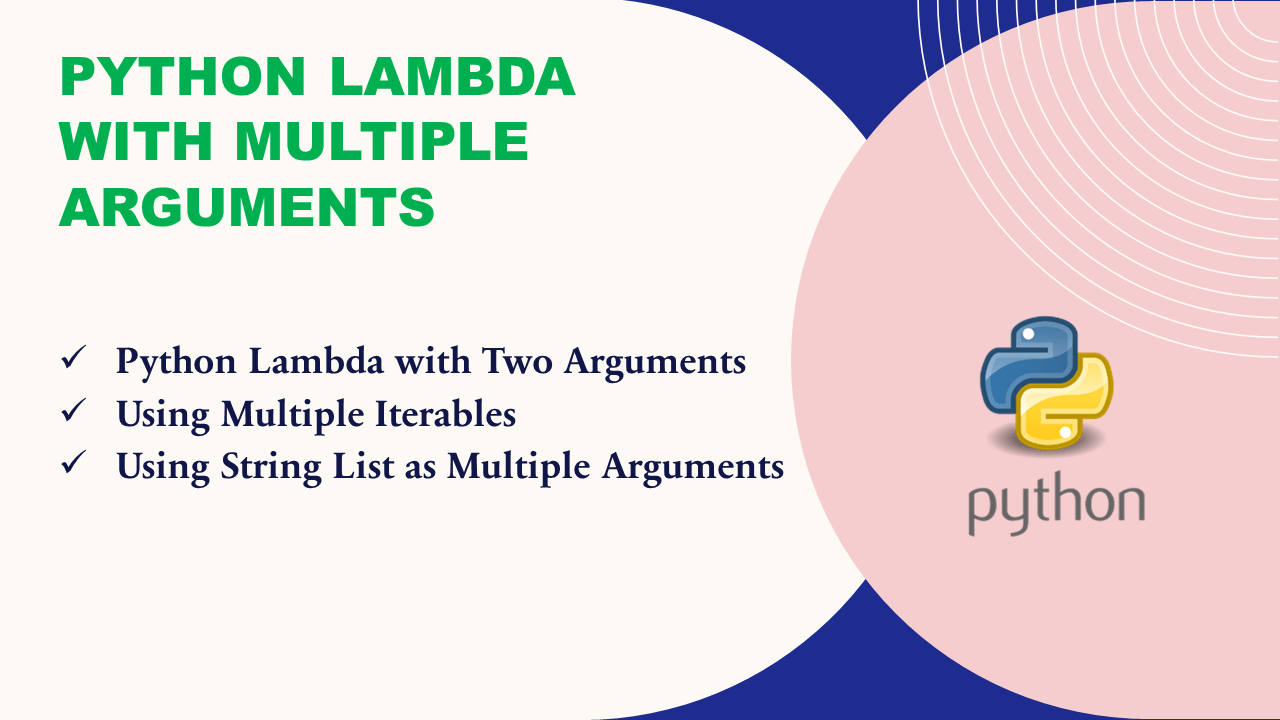# Python Lambda with Multiple Arguments

Python lambda can be used with multiple arguments and these arguments are used in evaluating an expression to return a single value. A Python lambda function is used to execute an anonymous function, an anonymous meaning function without a name. This function can take any number of arguments, but can only have one expression and they can be used wherever function objects are required. Here is the syntax of the lambda.

``````
# Syntax of lambda
lambda argument, [argument, argument]: expression
``````

Note that the expression in the lambda function body should return some value. If the expression does not return any value, the result from a lambda will be a `None` value.

Here is a simple example of lambda.

``````
# Lambda example with single argument
square = lambda x: x * x
print(square(4))

# Output:
# 16
``````

## 1. Python Lambda with Two Arguments

You can create a lambda function with multiple arguments in Python, let’s see an example with two arguments, add them and return the result. Here, I have created a add variable and assigned a lambda function to it.

Regardless of how many arguments you use with lambda, it always returns a single value. However, you can use the lambda function with map() to work on the iterable and get the iterable as a return type.

``````
# Lambda example with two arguments
add = lambda x, y : x + y

#Output:
#30
``````

Alternatively, you can also write the statement as follows. This is called inline execution. For inline invocation, we surround the lambda function with parentheses and place the values for the arguments next to it enclosed within parentheses.

``````
result = (lambda x, y : x + y)(10,20)
print(result)
``````

## 3. Using Multiple Iterables

Let’s create a lambda function with multiple arguments, and take the values for these arguments from the list, To pass values to these arguments you need to use two iterables.

``````
# Create two lists with numbers
numbers1 = [2, 4, 5, 6, 3]
numbers2 = [1, 3, 2, 2, 4]

# Create lambda function that takes two arguments
add_fun = lambda x, y: x+y

\$ Use lambda with map() function

# Output:
# [3, 7, 7, 8, 7]
``````

Here, the python lambda takes multiple arguments `x`, `y` and adds their values. The map() function applies this lambda function to each item of the `numbers1` & `numbers2` lists, and the result is a new iterator containing the sum of both numbers element-wise.

## 4. Using String List as Multiple Arguments

So far you have seen calling the custom functions, now let’s use the lower() function with lambda and map(). Here, I convert the list of string values to lowercase.

``````
# Create string list
myStrings1 = ["A","B","C","D"]
myStrings2 = ["a","b","c","d"]
print("myStrings1:",myStrings1)
print("myStrings2:",myStrings2)

# Use lower() function
lower_result = list(map(lambda x,y: x + y, myStrings1, myStrings2))
print("Result:",lower_result)

# Output:
# myStrings1: ['A', 'B', 'C', 'D']
# myStrings2: ['a', 'b', 'c', 'd']
# Result: ['Aa', 'Bb', 'Cc', 'Dd']
``````

## Conclusion

In this article, you have learned how to use python lambda with multiple arguments with examples. Also learned to use multiple iterable arguments with map() and lambda function.

## Related Articles

### Naveen

I am a Data Engineer with 20+ years of experience in transforming data into actionable insights. Over the years, I have honed my expertise in designing, implementing, and maintaining data pipelines with frameworks like Apache Spark, PySpark, Pandas, R, Hive and Machine Learning. My journey in the field of data engineering has been a continuous learning, innovation, and a strong commitment to data integrity. I have started this SparkByExamples.com to share my experiences with the data as I come across. You can learn more about me at LinkedIn